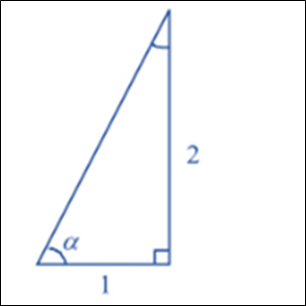# The remaining trigonometric ratios: tan α = 2 , 0 &lt; α &lt; π 2### Single Variable Calculus: Concepts...

4th Edition
James Stewart
Publisher: Cengage Learning
ISBN: 9781337687805### Single Variable Calculus: Concepts...

4th Edition
James Stewart
Publisher: Cengage Learning
ISBN: 9781337687805

#### Solutions

Chapter C, Problem 14E
To determine

## To calculate: The remaining trigonometric ratios:   tanα=2,   0<α<π2

Expert Solution

The remaining trigonometric ratios are listed as:

sinα=yr=25cosecα=ry=52cosα=xr=15secα=rx=51cotα=xy=12

### Explanation of Solution

Given information:

tanα=2,0<α<π2

Formula Used:

Trigonometric ratios are given by the following formula:

sinθ=yrcosecθ=rycosθ=xrsecθ=rxtanθ=yxcotθ=xy

Calculation:

From the given data:

tanα=yxtanα=2

So, y=2,x=1 the angle is constructed as below:Now, find the value of r

r=x2+y2r=12+42r=5

Therefore, remaining trigonometric ratios are calculated as:

sinα=yr=25cosecα=ry=52cosα=xr=15secα=rx=51cotα=xy=12

### Have a homework question?

Subscribe to bartleby learn! Ask subject matter experts 30 homework questions each month. Plus, you’ll have access to millions of step-by-step textbook answers!